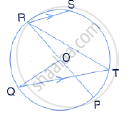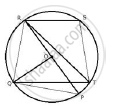Share

The Given Figure Shows a Circle with Centre O Such that Chord Rs is Parallel to Chord Qt, Angle Prt = 20° and Angle Poq = 100°. Calculate: (Ii) Angle Qrp - ICSE Class 10 - Mathematics

ConceptArc and Chord Properties - If Two Arcs Subtend Equal Angles at the Center, They Are Equal, and Its Converse

Question

The given figure shows a circle with centre O such that chord RS is parallel to chord QT, angle PRT = 20° and angle POQ = 100°. Calculate:

(ii) angle QRPSolutionJoin PQ, RQ and ST.

Arc QP subtends ∠QOPat the centre and ∠QRP at the remaining part of the circle.
∴  ∠QRP = 1/2  ∠QOP
⇒ ∠QRP = 1/2 xx 100° = 50°

Is there an error in this question or solution?

APPEARS IN

Solution The Given Figure Shows a Circle with Centre O Such that Chord Rs is Parallel to Chord Qt, Angle Prt = 20° and Angle Poq = 100°. Calculate: (Ii) Angle Qrp Concept: Arc and Chord Properties - If Two Arcs Subtend Equal Angles at the Center, They Are Equal, and Its Converse.
S